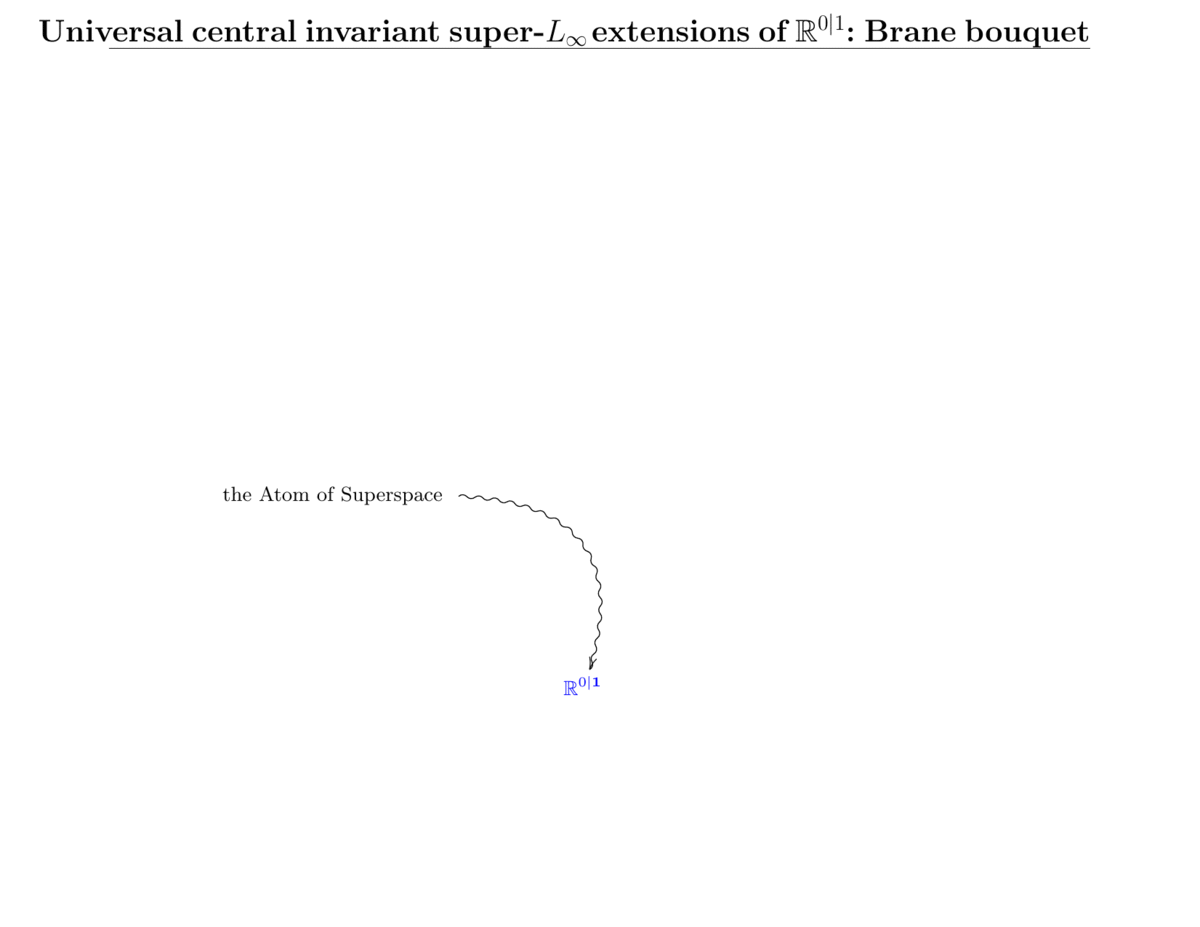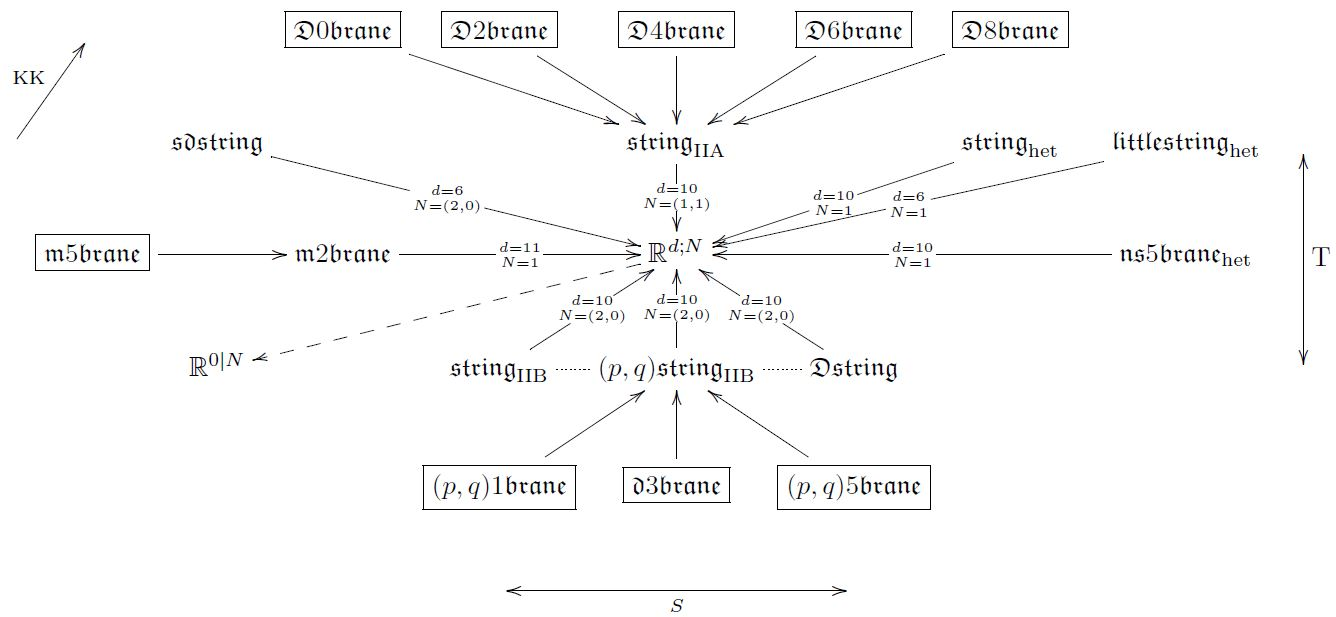# Schreiber The brane bouquet

Contents

An article that we have written:

• Super Lie $n$-algebra extensions, higher WZW models and super $p$-branes with tensor multiplet fields

International Journal of Geometric Methods in Modern Physics

Volume 12, Issue 02 (2015) 1550018 (35 pages)

arXiv:1308.5264

doi:10.1142/S0219887815500188

about ∞-Wess-Zumino-Witten theory for the brane scan of string/M-theory, in the context of supergeometric differential cohomology in a cohesive topos.

$\,$Followup articles:

Lecture notes

# Contents

## Abstract

We formalize higher dimensional and higher gauge WZW-type sigma-model local prequantum field theory, and discuss its rationalized/perturbative description in (super-)Lie n-algebra homotopy theory (the true home of the “FDA”-language used in the supergravity literature). We show generally how the intersection laws for such higher WZW-type sigma-model branes (open brane ending on background brane) are encoded precisely in (super-)$L_\infty$-extension theory and how the resulting “extended (super-)spacetimes” formalize spacetimes containing $\sigma$-model brane condensates. As an application we prove in Lie $n$-algebra homotopy theory that the complete super $p$-brane spectrum of superstring/M-theory is realized this way, including the pure sigma-model branes (the “old brane scan”) but also the branes with tensor multiplet worldvolume fields, notably the D-branes and the M5-brane. For instance the degree-0 piece of the higher symmetry algebra of 11-dimensional spacetime with an M2-brane condensate turns out to be the “M-theory super Lie algebra”. We also observe that in this formulation there is a simple formal proof of the fact that type IIA spacetime with a D0-brane condensate is the 11-dimensional sugra/M-theory spacetime, and of (prequantum) S-duality for type II string theory. Finally we give the non-perturbative description of all this by higher WZW-type $\sigma$-models on higher super-orbispaces with higher WZW terms in higher differential geometry.

## Seminar notes and Talk notes

Introductory seminar notes on the topic include the following:

Talk notes on the same topic include the following:Last revised on December 9, 2022 at 15:51:02. See the history of this page for a list of all contributions to it.